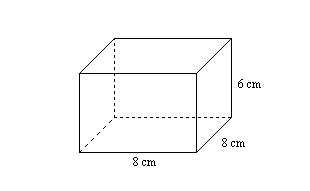Name:    Chapter 1 Test

Multiple Choice
Identify the choice that best completes the statement or answers the question.

Evaluate the expression when a = 5, b = 9, and c = 10.

1.

25 ¸ a
 a. 5 c. 6 b. 7 d. 20

2.

a + b
 a. 19 c. 14 b. 17 d. 15

3.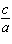a. 2 c. 4 b.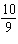d. 0

4.

a • c
 a. 49 c. 53 b. 50 d. 15

Evaluate the expression when a = 14, b = 10, and c = 8.

5.

2b –  4.6
 a. 16.4 c. 15.4 b. 12.4 d. 20

Write the phrase as an expression.

6.

10 less than 12
 a.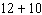c.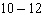b.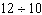d.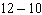7.

the total of 9 and 10
 a.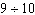c.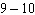b.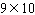d.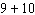8.

The expression 16 + x + 22 represents the perimeter of a triangle. Simplify the expression.
 a. 38 c. 54 b. x + 38 d. 38x

Use the Distributive Property to write and simplify an expression for the area of the rectangle.

9.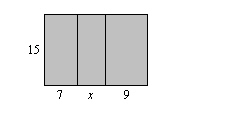a.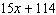c.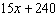b.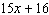d.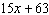Find the value of x that makes the expressions equivalent.

10.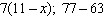a. 11 c. 9 b. 14 d. 7

11.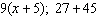a. 72 c. 3 b. 5 d. 9

Use the Distributive Property to rewrite the expression as a product.

12.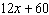a.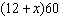c.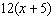b.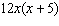d.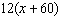Use a formula to find the area of the figure.

13.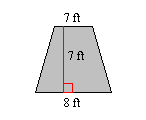a. 105 ft2 c. 52.5 ft2 b. 56 ft2 d. 28 ft2

14.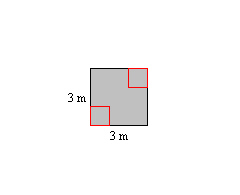a. 9 m2 c. 18 m2 b. 6 m2 d. 4.5 m2

Numeric Response

Use the Distributive Property and mental math to find the product.

1.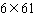Use a formula to find the area of the figure.

2.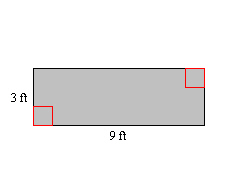3.

The formula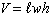represents the volume of a rectangular prism with length, width w, and height h. What is the volume of the rectangular prism?